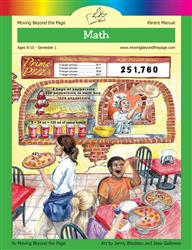# Common Core Alignment

CCSS.Math.Content.4.NBT.4 - Fluently add and subtract multi-digit whole numbers using the standard algorithm.

## 5: MathUnit 1: Place Value to 1,000,000
Lesson 10: Unit Test
Lesson 7: Adding and Subtracting Big Numbers
Lesson 8: Rounding to Estimate
Lesson 9: Problem Solving
Final Project: Cross Number PuzzleUnit 2: The Four Operations
Lesson 1: Addition Practice and Problem Solving
Lesson 14: Unit Test
Lesson 2: Subtraction Practice and Problem Solving